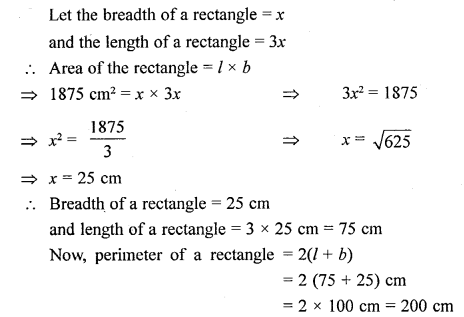## Selina Concise Mathematics Class 6 ICSE Solutions Chapter 32 Perimeter and Area of Plane Figures

Selina Publishers Concise Mathematics Class 6 ICSE Solutions Chapter 32 Perimeter and Area of Plane Figures

APlusTopper.com provides step by step solutions for Selina Concise ICSE Solutions for Class 6 Mathematics. You can download the Selina Concise Mathematics ICSE Solutions for Class 6 with Free PDF download option. Selina Publishers Concise Mathematics for Class 6 ICSE Solutions all questions are solved and explained by expert mathematic teachers as per ICSE board guidelines.

IMPORTANT POINTS

1. Perimeter: It is the length of the boundry of the given figure.
(i) Perimeter of a triangle = Sum of its three sides.
(ii) Perimeter of rectangle = 2 (length + breadth)
(iii) Perimeter of square = 4 x side.
2. Area: Area is the measure of surface of the plane covered by a closed plane figure. In other words, we can say that area of a closed plane figure is the measure of its interior region.
(i) Area of rectangle = length x breadth
(ii) Area of square = (side)².
3. Units of measurement of perimeter and area :
(i) Perimeter is measured in centimetre (cm) metre (m) or millimetre (mm).
(ii) Area is measured in square mm, square cm or square metre.

### Perimeter and Area of Plane Figures Exercise 32A – Selina Concise Mathematics Class 6 ICSE Solutions

Question 1.
What do you understand by a plane closed figure?
Solution:
Any geometrical plane figure bounded by lines (straight or curved) in a plane is called a plane closed figure.
Each of the following figures is a plane closed figure.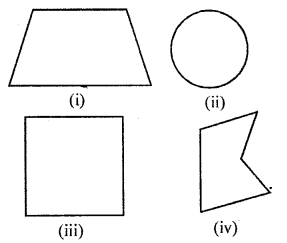Question 2.
The interior of a figure is called region of the figure. Is this statement true ?
Solution:
Yes. The interior of the figure alongwith its boundary is called region of the figure

Question 3.
Find the perimeter of each of the following closed figures :
Solution:
(i) Required perimeter
= AB + AC + BE + EF + FH + HG + HD
= 15 + 5 + 25 + 10 + 5 + 15 + 25 = 110 cm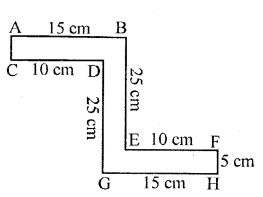(ii) Required perimeter
= AB + AC + CD + DG + BF + EF + EH + GH
= 20 + 4 + 8 + 20 + 4 + 8 + 20 + 4 = 88 cmQuestion 4.
Find the perimeter of a rectangle whose:
(i) length = 40 cm and breadth = 35 cm
(ii) length = 10 m and breadth = 8 m
(iii) length = 8 m and breadth = 80 cm
(iv) length = 3.6 m and breadth = 2.4 m
Solution:
(i) length = 40 cm and breadth = 35 cm
∴Perimeter = 2 (length + breadth)
= 2 (40 cm + 35 cm)
= 2 x 75 cm
= 150 cm = $$\frac { 150 }{ 100 }$$
= 1.5 m
(ii) length = 10 m and breadth = 8 m
∴Perimeter = 2 (length + breadth)
= 2 (10 m + 8 m)
= 2 x 18 m = 54 m
(iii) length = 8 m and
Length = 8 m
Breadth = 80 cm= $$\frac { 80 }{ 100 }$$ m = 0.8 m
∴ Perimeter = 2 (length + breadth)
= 2 (8 m + 0.8m)
= 2 x 8.8 m = 17.6 m
(iv) length = 3.6 m and breadth = 2.4 m
∴ Perimeter = 2 (length + breadth)
= 2 (3.6 m + 2.4 m)
= 2 x 6 m = 12 m

Question 5.
If P denotes perimeter of a rectangle, l denotes its length and b denotes its breadth, find :
(i) l, if P = 38cm and b = 7cm
(ii) b, if P = 3.2m and l = 100 cm
(iii) P, if l = 2 m and b = 75cm
Solution: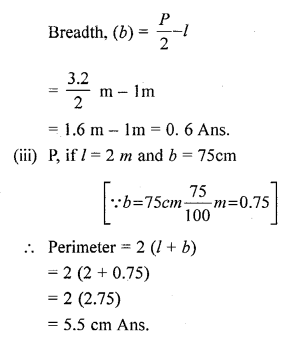Question 6.
Find the perimeter of a square whose each side is 1.6 m.
Solution:
∵ Side of the square = 1.6 m
∴ its perimeter = 4 x side
= 4 x 1.6 m
= 6.4 m

Question 7.
Find the side of the square whose pe-rimeter is 5 m.
Solution: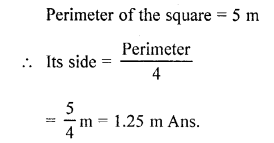Question 8.
A square field has each side 70 m whereas a rectangular field has length = 50 m and breadth = 40 m. Which of the two fields has greater perimeter and by how much?
Solution:
Perimeter of the square field = 4 x side = 4 x 70m = 280m
Perimeter of rectangular field = 2 (length + breadth)
= 2 (50 m + 40 m)
= 2 x 90 m
= 180 m
∴Square field has greater perimeter by 280 m – 180 m = 100 m

Question 9.
A rectangular field has length = 160m and breadth = 120 m. Find :
(i) the perimeter of the field.
(ii) the length of fence required to enclose the field.
(iii) the cost of fencing the field at the rate of ? 80 per metre.
Solution:
Given = length = 160 m, breadth = 120m
(i) The Perimeter of the field = 2 (l + b)
= 2 (160 m + 120 m)
= 2 x 280
= 560 m
(ii) The length of fence required to enclose the field = The perimeter of the rectan-gular field
= 560 m
(iii) The cost of fencing the field = Length of fence x Rate of fence
= 560 m x ₹80 per metre
= ₹44, 800

Question 10.
Each side of a square plot of land is 55 m. Find the cost of fencing the plot at the rate of ₹32 per metre.
Solution:
∵Perimeter of square field = 4 x its side = 4 x 55 m
∴Length of required fencing = 220 m Now, the cost of fencing = its length x its rate
= 220 m x ₹32 per metre?
= ₹7040

Question 11.
Each side of a square field is 70 cm. How much distance will a boy walk in order to make ?
(i) one complete round of this field ?
(ii) 8 complete rounds of this field ?
Solution:
(i) Distance covered by the boy to make one complete round of the field.
Perimeter of the field : 4 x its side = 4 x 70 = 280 m
(ii) Distance covered by the boy to make 8 complete rounds of this field.
= 280 m x 8 m = 2240 m

Question 12.
A school playground is rectangular in shape with length = 120 m and breadth = 90 m. Some school boys run along the boundary of the play-ground and make 15 complete rounds in 45 minutes. How much distance they run during this period.
Solution:
Length of the rectangular playground = 120 mBreadth of the rectangular playground = 90 m
∴ Perimeter of the rectangular ground = 2(l + b)
= 2(120 + 90) m = 420 m
Thus, in one complete round, boys covers a distance of = 420 m
∴Distance covered in 15 complete rounds = 420 m x 15 = 6300 m

Question 13.
Mohit makes 8 full rounds of a rect-angular field with length = 120 m and breadth = 75 m.
John makes 10 full rounds of a square field with each side 100 in. Find who covers larger distance and by how much?
Solution:
Mohit
Length of the rectangular field = 120
Breadth of the rectangular field = 75 m
∴ Distance covered in one round (perim-eter) = 2(1 + b)
= 2(120 + 75) = 390 m Hence, distance covered in 8 rounds = 390 x 8 m = 3120 m
John
Side of the field = 100 m
∴Distance covered in one round = 4 x a = 4 x 100 = 400 m
Hence, Distance covered in 10 rounds = 400 x 10 m = 400 m
John a covers greater distance then Mohit by = (4000-3120) m = 880 m

Question 14.
The length of a rectangle is twice of its breadth. If its perimeter is 60 cm, find its length.
Solution:
Let the breadth of the field = x cm
∴ its length = 2x
and, its perimeter = 2 x (length + breadth)
= 2 x (2x + x)
= 2(3x)
= 6x cm
Perimeter = 60 cm
⇒ 60 cm = 6x cm
⇒ x = $$\frac { 60 }{ 6 }$$ = 10 cm
∴Breadth = x = 10 cm
Length = 2x = 2 x 10 = 20 cm

Question 15.
Find the perimeter of :
(i) an equilateral triangle of side 9.8 cm.
(ii) an isosceles triangle with each equal side = 13 cm and the third side = 10 cm.
(iii) a regular pentagon of side 8.2 cm.
(iv) a regular hexagon of side 6.5 cm.
Solution:
(i) The perimeter of equilateral triangle = 3 x side
= 3 x 9.8 cm
= 29.4 cm
(ii) Required perimeter = 13 cm + 13 cm + 10 cm
= 36 cm
(iii) Perimeter of given pentagon = 5 x side = 5 x 8.2 cm
= 41 cm
(iv) Perimeter of given hexagon = 6 x side = 6 x 6.5 cm
= 39 cm

Question 16.
An equilateral triangle and d square has equal perimeter. If side of the triangle is 9.6 cm ; what is the length of the side of the square ?
Solution:
Perimeter of equilateral triangle = Perimeter of square Side of triangle = 9.6 cm
∴Perimeter of triangle = 3 x side
= 3 x 9.6 cm = 28.8 cm
> Perimeter of the square = 28.8 cm
4 x the side of square = 28.8 cm
⇒ The side of the square = $$\frac { 28.8 }{ 4 }$$ cm
= 7.2 cm Ans.

Question 17.
A rectangle with length = 18 cm and breadth = 12 cm has same perimeter as that of a regular pentagon. Find the side of the pentagon.
Solution:
Length of rectangle = 18 cm
Breadth of rectangle = 12 cm
∴ Perimeter of rectangle = 2 x (l + b)
= 2 x (18+12)
= 2 x 30 = 60 cm
∵Perimeter, of rectangle = Perimeter of pentagon
60 cm = 5 x side
side = $$\frac { 60 }{ 5 }$$ cm = 12 cm
∴Side of the pentagon = 12 cm Ans.

Question 18.
A regular pentagon of each side 12 cm has same perimeter as that of a regular hexagon. Find the length of each side of the hexagon.
Solution:
Perimeter of regular pentagon = 5 x length of the side
= 5 x 12 cm = 60 cm
Clearly, perimeter of the given pentagon = 60 cm
⇒ 6 x side of hexagon = 60 cm 60
⇒ side of hexagon = $$\frac { 60 }{ 6 }$$cm = 10 cm

Question 19.
Each side of a square is 45 cm and a rectangle has length 50 cm. If the perimeters of both (square and rectangle) are same, find the breadth of the rectangle.
Solution: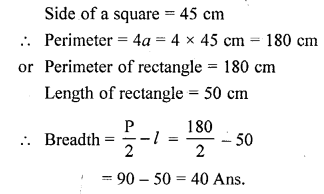Question 20.
A wire is bent in the form of an equilateral triangle of each side 20 cm. If the same wire is bent in the form of a square, find the side of the square.
Solution:
∵Each side of the given equilateral triangle = 20 cm
∴Perimeter of the triangle = 3 x side = 3 x 20 cm = 60 cm ,
∴ Perimeter of the square = Perimeter of equilateral triangle
⇒ 4 x side of square = 60 cm
⇒ The side of the square = $$\frac { 60 }{ 4 }$$
=15 cm

### Perimeter and Area of Plane Figures Exercise 32B – Selina Concise Mathematics Class 6 ICSE Solutions

Question 1.
Find the area of a rectangle whose :
(i) length = 15 cm breadth = 6.4 cm
(ii) Length = 8.5 m breadth = 5 m
(iii) Length = 3.6 m breadth = 90 cm
(iv) Length = 24 cm breadth =180 mm
Solution:Question 2.
Find the area of a square, whose each side is :
(i) 7.2 cm
(ii) 4.5 m
(iii) 4.1 cm
Solution:
(i) 7.2 cm
Area of the square = (side)² = (7.2 cm)² = 7.2 cm x 7.2 cm = 51.84 cm²
(ii) 4.5 m
Area of the square = (side)² = (4.5 m)² = 4.5 m x 4.5 m = 20.25 m²
(iii) 4.1 cm
Area of the square = (side)² = (4.1 cm)² = 4.1 cm x 4.1 cm = 16.81 cm²

Question 3.
If A denotes area of a rectangle, l represents its length and b represents its breadth, find :
(i) l, if A = 48 cm² and b = 6 cm
(ii) b, if A = 88 m² and l = 8m
Solution:Question 4.
Each side of a square is 3.6 cm; find its
(i) perimeter
(ii) area.
Solution:
(i) Perimeter = 4 x side
= 4 x 3.6 cm = 14.4 cm
(ii) Area = (side)²
= (3.6 cm)²
= 12.96 cm²

Question 5.
The perimeter of a square is 60 m, find :
(i) its each side its area
(ii) its new area obtained on increasing
(iii) each of its sides by 2 m.
Solution:
Perimeter of a square = 60 m
(i) Perimeter of a square = 4 x side
60 m = 4 x side
$$\frac { 60 }{ 4 }$$ = side 4
∴side = 15 m
(ii) Area of square = (side)² = (15 m)²
= 15 m x 15 m
= 225 m²
(iii) Increased each side = 2 m
Side of square = 15 m
New length of side = (2m + 15m)
= 17m
∴New Area of square = (17m)² = 17m x 17m = 289 m²

Question 6.
Each side of a square is 7 m. If its each side be increased by 3 m, what will be the increase in its area.
Solution:
Each side of square = 7 m
∴Area of square = (side)²= (7 m)²
= 7m x 7m =49m²
∵ Side increased by 3 m
∴Total length of side will be = 3 m + 7 m = 10m
∴Area of square = (10 m)²= 10m x 10 m = 100 m²
∴Increase in area = 100 m² – 49 m² = 51 m²

Question 7.
The perimeter of a square field is numerically equal to its area. Find each side of the square.
Solution:Question 8.
A rectangular piece of paper has area = 24 cm² and length = 5 cm. Find its perimeter.
Solution:Question 9.
Find the perimeter of a rectangle whose area = 2600 m² and breadth = 50 m.
Solution: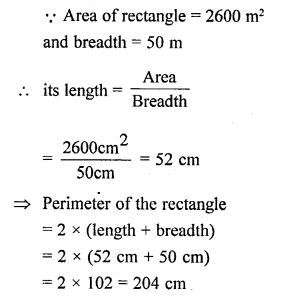Question 10.
What will happen to the area of a rectangle, if its length and breadth both are trebled?
Solution:
Let the original length of the rectangle = l and its original breadth = b
∴ its original area = length x breadth i.e A = l – b i. e.
Since,
Increased length -=3l
∴ New area = 3l x 3b = 9 x l x b [∵A = l x b]
⇒ Area of the new rectangle = 9 times than area of original rectangle

Question 11.
Length of a rectangle is 30 m and its breadth is 20 m. Find the increase in its area if its length is increased by 10 m and its breadth is doubled.
Solution:
Length of a rectangle (l) = 30 m,
Breadth of the rectangle (b) = 20 m
Area of rectangle = l x b
= 30 x 20 = 600 m2
Since, the length its increased by 10 m and breadth is doubled
∴New length (l) = (30 + 10) m = 40 m
and new breadth = (20 x 2) m = 40 m
∴New area = l x b = 40 x 40 m2 = 1600 m2
Hence, the increase in the area = (1600 – 600) m2
= 1000 m2

Question 12.
The side of a square field is 16 m. What will be increase in its area, if:
(i) each of its sides is increased by 4 m
(ii) each of its sides is doubled.
Solution: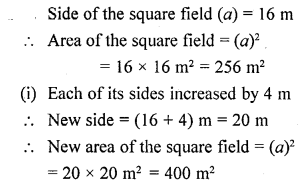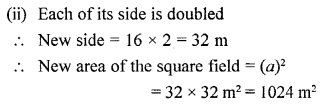Question 13.
Each rectangular tile is 40 cm long and 30 cm wide. How many tiles will be required to cover the floor of a room with length = 4.8 m and breadth = 2.4 m.
Solution: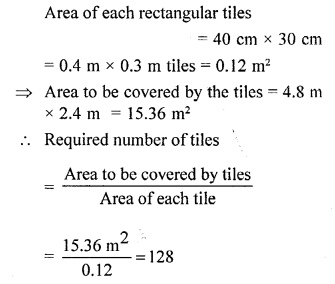Question 14.
Each side of a square tile is 60 cm. How many tiles will be required to cover the floor of a hall with length = 50 m and breadth = 36 m.
Solution: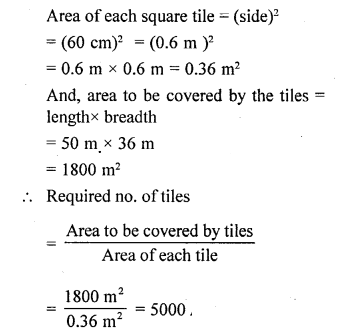Question 15.
The perimeter of a square plot = 360 m. Find :
(i) its area.
(ii) cost of fencing its boundary at the rate of ₹ 40 per metre.
(iii) cost of levelling the plot at ₹60 per square metre.
Solution:
Given, perimeter of square plot = 360 m
∵ Perimeter of the square = 4 x its side
∴4 x side of square = 360 m
⇒ side of the square = $$\frac { 360m }{ 4 }$$ = 90 m
(i) The area of the square field = (side)²
= (90 m)²
= 90 m x 90 m
= 8100 m²
Cost of fencing at ₹ 40 per metre
= 8100 m2 x ₹ 40 per metre
= ₹ 324000
Cost of levelling at₹ 60 per m²
= 8100 m² x ₹60 per m²
= ₹ 486000

Question 16.
The perimeter of a rectangular field is 500 m and its length = 150 m. Find:
(ii) its area.
(iii) cost of ploughing the field at the rate of ₹1.20 per square metre.
Solution:
(i) Perimeter of a rectangle = 2 x (length + breadth)
⇒500 m = 2x(i50m + breadth)
⇒250 m – 150 m = breadth
(ii) Area of rectangular field = length x breadth
= 150 m x 100 m = 15000 m²
(iii) Cost of ploughing the field at the rate of
= ₹1.20 per square m²= area of the field x rate of ploughing = 15000 m² x ₹1.20 per square metre = ₹15000 x 1.20 = ₹18000

Question 17.
The cost of flooring a hall of ₹64 per square metre is ₹2,048. If the breadth of the hall is 5m, find :
(i) its length.
(ii) its perimeter.
(iii) cost of fixing a border of very small width along its boundary at the rate of ₹60 per square metre.
Solution: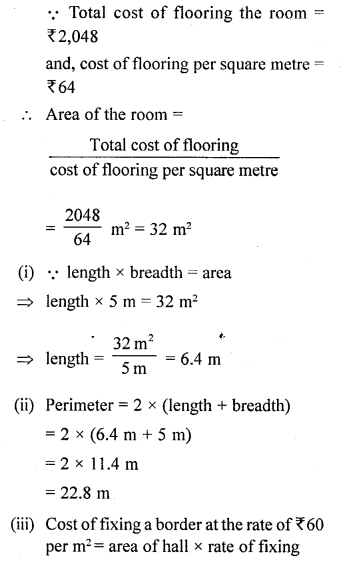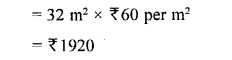Question 18.
The length of a rectangle is three times its breadth. If the area of the rectangle is 1875 sq. cm, find its perimeter.
Solution: